# ML Aggarwal Class 6 Solutions for ICSE Maths Chapter 4 Playing with Numbers Objective Type Questions

## ML Aggarwal Class 6 Solutions for ICSE Maths Chapter 4 Playing with Numbers Objective Type Questions

Mental Maths
Question 1.
Fill in the blanks:
(i) The only natural number which has exactly one factor is ……….
(ii) The only prime number which is even is ……….
(iii) The HCF of two co-prime numbers is ……….
(iv) Two perfect numbers are ………. and ……….
(v) The only prime-triplet is ……….
Solution:Question 2.
State whether the following statements are true (T) or false (F):
(i) Every natural number has a finite number of factors.
(ii) Every natural number has an infinite number of its multiples.
(iii) There are infinitely many prime numbers.
(iv) If two numbers are separately divisible by a number, then their difference is also divisible by that number.
(v) LCM of two prime numbers equals their product.
(vi) LCM of two co-prime numbers equals their product.
Solution:Question 3.
State whether the following statements are true or false. If a statement is false, justify your answer.
(i) The sum of two prime numbers is always an even number.
(ii) The sum of two prime numbers is always a prime number.
(iii) The sum of two prime numbers can never be a prime number
(iv) No odd number can be written as the sum of two prime numbers.
(v) If two numbers are co-prime, then atleast one of them must be prime.
(vi) If a number is divisible by 18, it must be divisible by 3 and 6 both.
(vii) If a number is divisible by 2 and 4 both, it must be divisible by 8.
(viii) If a number is divisible by 3 and 6 both, it must be divisible by 18.
(ix) HCF of an even number and an odd number is always 1.
Solution: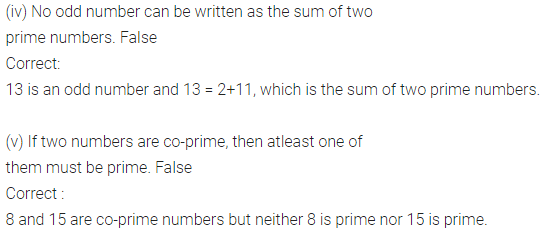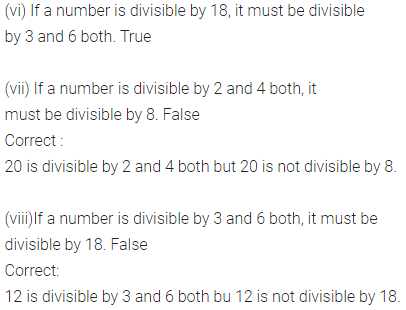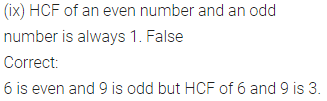Multiple Choice Questions
Choose the correct answer from the given four options (4 to 28):
Question 4.
All factors of 6 are
(a) 1, 6
(b) 2, 3
(c) 1, 2, 3
(d) 1, 2, 3, 6
Solution:Question 5.
Which of the following is an odd composite number?
(a) 7
(b) 9
(c) 11
(d) 12
Solution:Question 6.
The number of even numbers between 68 and 90 is
(a) 10
(b) 11
(c) 12
(d) 31
Solution:Question 7.
Which of the following is a prime number?
(a) 69
(b) 87
(c) 91
(d) 97
Solution:Question 8.
Which of the following is a pair of twin- prime number?
(a) 19, 21
(b) 43, 47
(c) 59, 61
(d) 73, 79
Solution:Question 9.
The number of distinct prime factors of the largest 4-digit number is
(a) 2
(b) 3
(c) 5
(d) none of these
Solution:Question 10.
The number of distinct prime factors of the smallest 5-digit number is
(a) 2
(b) 4
(c) 6
(d) 8
Solution:Question 11.
The sum of the prime factors of 1729 is
(a) 13
(b) 19
(c) 32
(d) 39
Solution: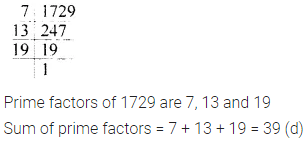Question 12.
Which of the following is a pair of co-prime numbers?
(a) 8, 45
(b) 3, 18
(c) 5, 35
(d) 6, 39
Solution:Question 13.
Every natural number has an infinite number of
(a) prime factors
(b) factors
(c) multiples
(d) none of these
Solution: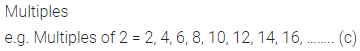Question 14.
Which of the following numbers is divisible by 4?
(a) 308594
(b) 506784
(c) 732106
(d) 9301538
Solution:Question 15.
Which of the following numbers is divisible by 8?
(a) 503786
(b) 505268
(c) 305678
(d) 703568
Solution: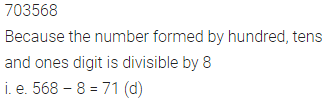Question 16.
Which of the following numbers is divisible by 3?
(a) 50762
(b) 42063
(c) 52871
(d) 37036
Solution: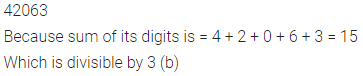Question 17.
Which of the following numbers is divisible by 9?
(a) 972063
(b) 730542
(c) 785423
(d) 5612844
Solution: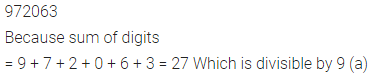Question 18.
Which of the following numbers is divisible by 6?
(a) 560324
(b) 650374
(c) 798653
(d) 750972
Solution: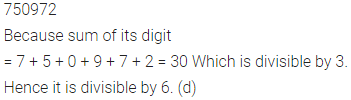Question 19.
The digit by which ‘*’ should be replaced in 54 * 281 so that the number formed is divisible by 9 is
(a) 6
(b) 7
(c) 8
(d) 9
Solution: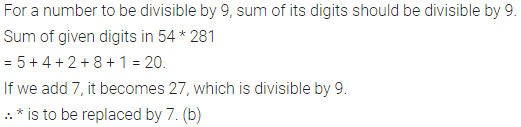Question 20.
The digit by which should be replaced in 7254 * 98 so that the number formed is divisible by 22 is
(a) 0
(b) 1
(c) 2
(d) 6
Solution:Question 21.
If a number is divisible by 5 and 6 both, then it may not be divisible by
(a) 10
(b) 15
(c) 30
(d) 60
Solution: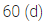Question 22.
The number of common prime factors of 60, 75 and 105 is
(a) 2
(b) 3
(c) 4
(d) 5
Solution: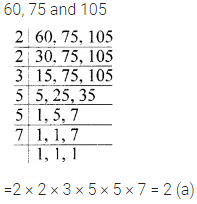Question 23.
The H.C.F. of 144 and 198 is
(a) 6
(b) 9
(c) 12
(d) 18
Solution:Question 24.
The L.C.M. of 30 and 45 is
(a) 15
(b) 30
(c) 45
(d) 90
Solution: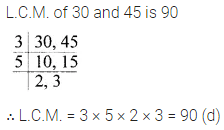Question 25.
The L.C.M. of 4 and 44 is
(a) 4
(b) 11
(c) 44
(d) 176
Solution:Question 26.
The LCM of 7 and 13 is 1
(a) 1
(b) 7
(c) 13
(d) 91
Solution: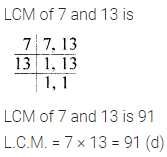Question 27.
If H.C.F. of two numbers is 15 and their product is 1575, then their L.C.M. is
(a) 15
(b) 105
(c) 525
(d) 1575
Solution:Question 28.
If the LCM of two natural numbers is 180, then which of the following is not the HCF of the numbers?
(a) 45
(b) 60
(c) 75
(d) 90
Solution: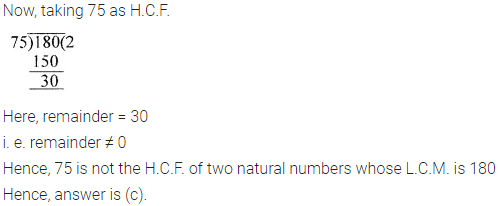Value Based Questions
Question 1.
To teach the value of gratitude and appreciation to the students, a school organised a ‘Card Making’ activity in which the students were asked to make “THANK YOU CARDS” for the people who helped them in some way. Assorted cards were made with different titles. Their numbers are given below:
T cards for teachers = 120
F cards for friends = 540
S cards for servants = 90
P cards for parents = 240 and
G cards for grandparents = 150
(i) Find the HCF and LCM of all the different number of cards.
(ii) Find HCF and LCM of maximum and minimum number of cards.
(iii) Is the number of T-cards is a factor of number of P-cards?
Solution: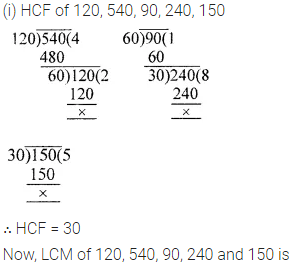Higher Order Thinking Skills (Hots)
Question 1.
Write 2-digit odd numbers whose sum of digits is 8.
Solution: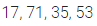Question 2.
Write all pairs of 2-digit twin primes such that on changing the places of their digits, they still remain prime numbers.
Solution: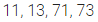Question 3.
There are just four natural numbers less than 100, which have exactly three factors. One of them is 25, what are the other three? What can be said about these numbers?
Solution: